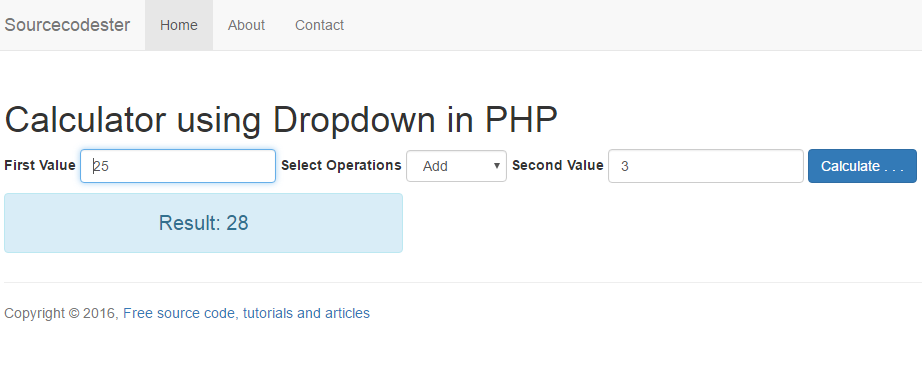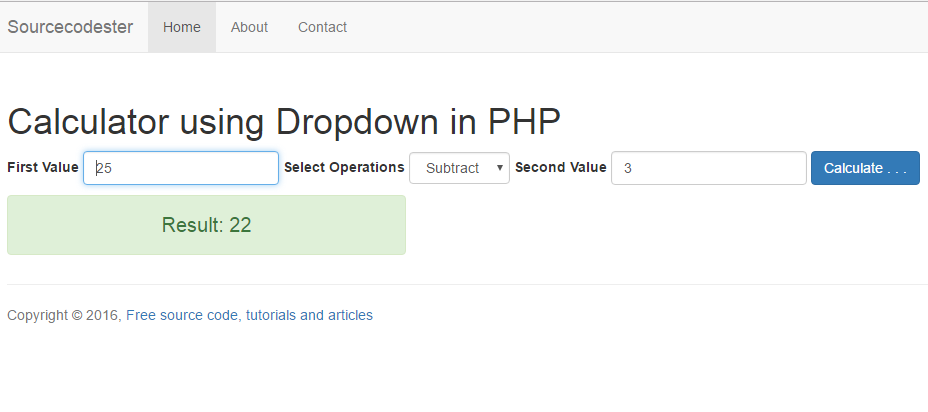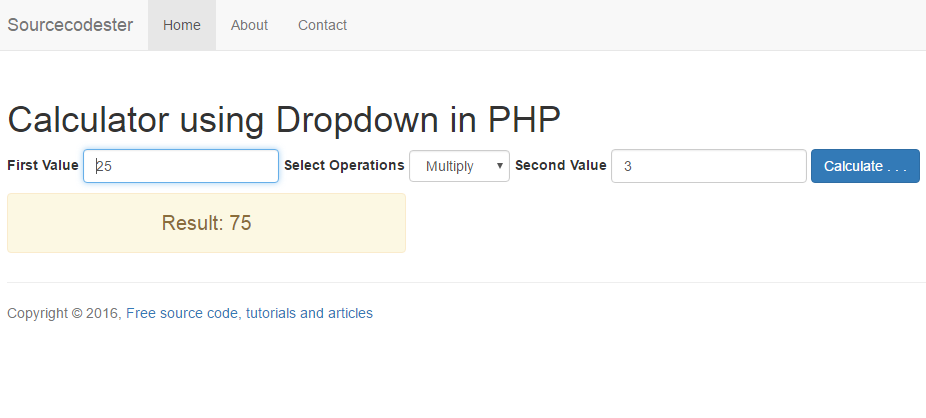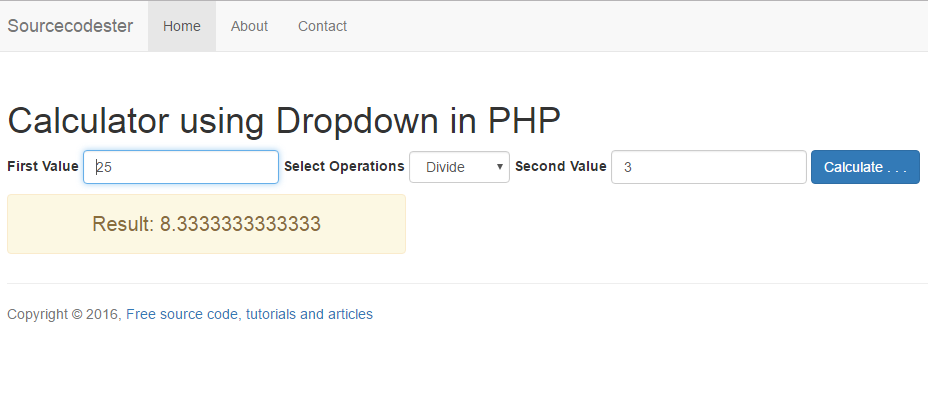# Calculator using Dropdown in PHP

In this tutorial, we are going to create Calculator using Dropdown in PHP. I create this simple program in PHP using the drop-down menu to select a mathematical operator such as Addition, Subtraction, Multiplication, and Division. Check the live demo, click the button below. First, we have to create the markup for this program. This source code contains two TextBox where the user enters the desired numeric value and one combo box where they can select in what mathematical operator they have to use.
To execute the calculator, we need to construct the PHP query where the operators can run after hit the calculate button on the web page. Let's create one by one. For Addition
Sample Output for AdditionFor Subtraction
Sample Output for SubtractionFor Multiplication
Sample Output for MultiplicationFor Division
Sample Output for DivisionFor the complete source code. Using the source code below, you can use a mathematical operator such as Addition, Subtraction, Multiplication, and Division.

Note: Due to the size or complexity of this submission, the author has submitted it as a .zip file to shorten your download time. After downloading it, you will need a program like Winzip to decompress it.

Virus note: All files are scanned once-a-day by SourceCodester.com for viruses, but new viruses come out every day, so no prevention program can catch 100% of them.